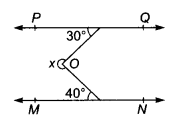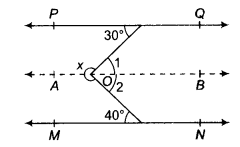# In the given figure, PQ \ \ MN. Find the value of x

In the given figure, PQ \ \ MN. Find the value of x.Through O, draw AB || PQ || MN∵ AB || PQ
∴ ∠1 = 30°
also,AB|| MN
then ∠2= 40° [alternate interior angles]
Now,∠x+∠1+∠2=360°[angles around a point]
⇒ x=360°-(∠1+∠2)
⇒ x = 360°-(30°+40°)
⇒ x = 290°